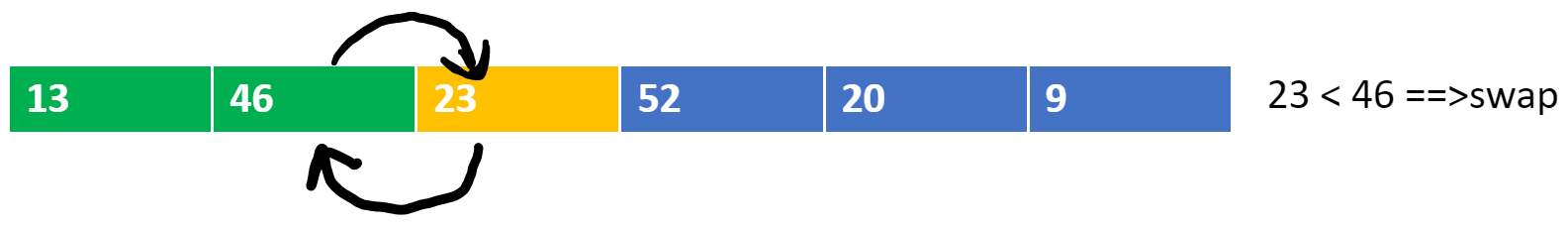# Insertion Sort Algorithm

Problem Statement: Given an array of N integers, write a program to implement the Insertion sorting algorithm.

Examples:

```Example 1:
Input: N = 6, array[] = {13,46,24,52,20,9}
Output: 9,13,20,24,46,52
Explanation:
After sorting the array is: 9,13,20,24,46,52

Example 2:
Input: N=5, array[] = {5,4,3,2,1}
Output: 1,2,3,4,5
Explanation: After sorting the array is: 1,2,3,4,5
```

### Solution

Disclaimer: Don’t jump directly to the solution, try it out yourself first.

Approach

• Take an element from the unsorted array.
• Place it in its corresponding position in the sorted part.
• Shift the remaining elements accordingly.
• In this approach, start iterating the “outer for loop”  from the 2nd position of the array.
• The “inner while loop” shifts the elements using swapping.
• In the below dry run,

Blue color represents unsorted array elements

The yellow color represents the current element to be placed at the appropriate position.

The green color represents the sorted array elementsCode:

## C++ Code

``````#include<bits/stdc++.h>

using namespace std;
void insertion_sort(int arr[], int n) {
// insertion sort
for (int i = 1; i < n; i++) {
int current = arr[i];
int j = i - 1;
while (j >= 0 && arr[j] > current) {
//swap
arr[j + 1] = arr[j];
j--;
}
arr[j + 1] = current;
}
cout << "After insertion sort:" << "\n";
for (int i = 0; i < n; i++) {
cout << arr[i] << " ";
}
cout << "\n";

}
int main() {
int arr[] = {13,46,24,52,20,9};
int n = sizeof(arr) / sizeof(arr);
cout << "Before insertion sort:" << "\n";
for (int i = 0; i < n; i++) {
cout << arr[i] << " ";
}
cout << "\n";
insertion_sort(arr, n);
return 0;
}
``````

Output:

Before insertion sort:
13 46 24 52 20 9
After insertion sort:
9 13 20 24 46 52

Time Complexity: O(n^2) (nested loops)

Space Complexity: O(1)

## Java Code

``````public class insertion_sort {
public static void main(String args[]) {
int arr[] = {13,46,24,52,20,9};
int n = arr.length;
System.out.println("Before insertion sort:");
for (int i = 0; i < n; i++) {
System.out.print(arr[i] + " ");
}
System.out.println();
//insertion sort
for (int i = 1; i < n; i++) {
int current = arr[i];
int j = i - 1;
while (j >= 0 && arr[j] > current) {
//swap
arr[j + 1] = arr[j];
j--;
}
arr[j + 1] = current;
}

System.out.println("After insertion sort:");
for (int i = 0; i < n; i++) {
System.out.print(arr[i] + " ");
}
System.out.println();

}

}
``````

Output:

Before insertion sort:
13 46 24 52 20 9
After insertion sort:
9 13 20 24 46 52

Time Complexity: O(n^2) (nested loops)

Space Complexity: O(1)

Special thanks to Rishiraj Girmal for contributing to this article on takeUforward. If you also wish to share your knowledge with the takeUforward fam, please check out this article